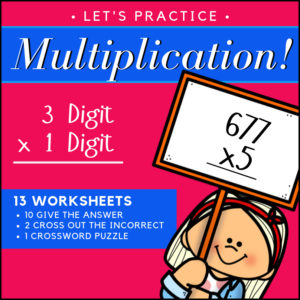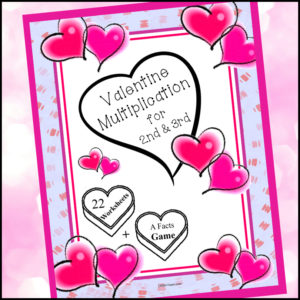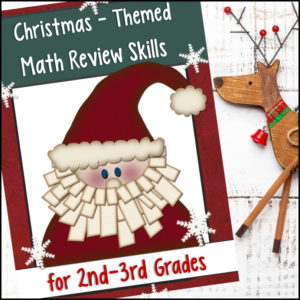Showing 1–20 of 29 results

•\$3.00

This resource is designed to give students the practice the need to become proficient at multiplying decimals. There are three levels, 15 worksheets (16 problems each) and answer keys. That’s 240 problems – EACH WORKSHEET includes a different growth mindset quote!
(See description below for more details)

•\$3.00

This resource is designed to give students the practice the need to become proficient at multiplying decimal numbers. There are three levels, 15 worksheets (20 problems each) and answer keys. That’s 300 problems! Use as practice sheets, homework, or for quizzes and tests.

Also included: Visual poster to help students with ‘where to place’ the decimal point in their answers.

3 Levels:

• Level 1 (5 worksheets)
Tenths multiplied by tenths
• Level 2 (5 worksheets)
Tenths multiplied by hundreds and/or by thousandths
Hundredths multiplied by hundredths and/or by thousandths
• Level 3 (5 worksheets)
Mixed from tenths/hundredths/thousandths multiplied by tenths/hundredths/thousandths
•\$2.25
•\$3.99

Students love playing games! Have them practice their multiplication knowledge and reinforce those Math facts with this FUN card game! It is themed for February – Valentine’s Day but can be used anytime of the year because students will love the hearts and penguins. Multiplication facts 0-10 are included.

Game Pieces

• – 96 math cards
• – 2 wild cards
• – Cover for the back of each card

Detailed game ‘HOW TO PLAY’ instructions included!

•\$4.99

Give your students a fun way to practice their multiplication facts! Your students will love this fast paced math game with included fun twists such as: the ‘Green Horn‘, ‘Stampede!’, ‘Playing in the Mud‘, ‘Stomped‘ and ‘Growing Herd‘. Your students are going to want to play this game again and again!

Includes:

• – Game instructions
• – Game Cards (all facts 0-12 PLUS fun ‘twist’ game cards)
• – (Optional) Card backs
•\$2.25

These 300 problems will give your students the practice they need to reinforce their multiplication skills (single digit number x double digit)! This resource includes 10 worksheets with 30 problems each. Each can be used by students for practice or for assessment (quiz and test). Answer keys provided.

Skill: Students will multiply a two digit whole number by a one-digit whole number.

•\$4.99

OVER 1,800 problems, your students will have the practice they need to understand and learn their multiplication facts 0 to 10!

The resource begins with repeated addition (to reinforce the concept of addition in relation to multiplication) and ends with students completing all multiplication facts.

•\$3.99

Here is a Valentine’s Day themed Multiplication for 2nd and 3rd Grades! Your students will love completing the single digit multiplication problems wrapped in holiday designs. You’ll find in this resource worksheets that will show both the addition aspect of multiplication visually represented as well as pages with only the multiplication equations.

These pages will help students…understand multiplication as well as practice and reinforce their knowledge of facts.

Also included: Valentine’s theme multiplication facts game!

•\$3.00

Help students learn and practice the basic concept behind multiplication with these 280 visual model problems. Designed for 2nd – 3rd Grades, students will demonstrate the ability to…

• – Determine the quantity of shapes shown
• – Create equations from arrays
• – Create arrays from given equations

Why arrays? A multiplication array is simply an arrangement of rows or columns that matches a multiplication equation. Students can more readily develop an understanding of multiplication concepts when they see visual representations of the computation process. Arrays are also the easiest and most logical way to show kids the commutative property of multiplication.

Included: 30 worksheets + answer keys.

•\$2.25

These 300 problems will give your students the practice they need to reinforce their multiplication skills (double digit x double digit)! This resource includes 15 worksheets with 20 problems each. Each can be used by students for practice or for assessment (quiz and test). Answer keys provided.

Skill: Students will multiply a double digit whole number by a double-digit whole number.

•\$2.00

Give your students the practice they need to reinforce and demonstrate fluency in multiplication with factors 0-12. This resource includes 200 problems – 10 worksheets with 20 problems each.

You can use as ‘normal’ worksheets or use in timed drills! Answer keys provided.

•\$3.00

These 500 problems will give your students the practice they need to reinforce their multiplication skills (triple digit x single – double – triple digit)! Can be used by students for practice or for assessment (quiz and test). Answer keys provided.

Skill: Students will multiply a triple digit whole number by a single, double or triple digit whole number.

Includes:

• – 5 Worksheets (triple x single digit) 125 problems total
• – 5 Worksheets (triple x double digit) 100 problems total
• – 5 Worksheets (triple x triple digit) 75 problems total
• – 5 Worksheets (mixed – triple x single, double and triple digit) 75 problems total
•\$2.00

Give to students so they can practice and reinforce multiplication skills and you to assess their knowledge!

Includes 11 spring themed worksheets:

• – Worksheets 1 – 9 are centered around one fact each…
(i.e page 1 covers x1 ; page 2 covers x2 ; page 9 covers x9s)
• – Worksheets 10 – 11 are fact family worksheets where students will address both multiplication and division problems of one fact (see example below)…
___ x 7 = 21
7 x __ = 21
21 ÷ 7 = __
__÷ 3 = 7
•\$8.00

This 38 page math glossary has been designed for 3rd-5th grades and includes 129 math terms with definitions and 72 illustrations! Terms include those surrounding basic operations, geometry and elementary statistics.

(See description below for a complete list of included terms)

•\$1.50
This Math resource includes cards for counting – by 1s, 2s, 3s, 5s and 10s and designed to help students develop number sense knowledge. Students can use to count and skip count. Can also be used to help students begin to understand multiplication concepts. Colorful and butterfly themed. Simply print, laminate and cut out individual cards to use again and again.

Preparation is low. Simply print, laminate and cut out. Use in Math centers or give each student their very own copies to use at their desk or at home.
•\$8.00

This February NO PREP packet is everything you’ll need for an entire month of Math review for 2nd and 3rd grades! Jam packed with a variety of activities to get your students practicing and reinforcing important Math skills: addition, subtraction, multiplication, word problems, critical thinking, geometry, measurement, time, fractions and more! JUST PRINT AND GO.

•\$3.00

This Math center resource has a St. Patrick’s Day theme and provide students with the ability to practice addition, subtraction, early multiplication/division, time, money, shape recognition, number patterns skills! Includes: 64 task cards, Math Center sign, student worksheet and an answer key.

** Critical thinking skills will definitely be used! **

•\$3.00

This resource can be used for hours of Math learning, reinforcement and practice during December and give lessons a Christmas holiday twist!

What you get:

• * Nutcracker themed number cards 1 – 120
• * Extra blank cards
• * 100s chart poster and handout
••